# Solve by factoring Solve by factoring Solve by

• Slides: 16Solve by factoring.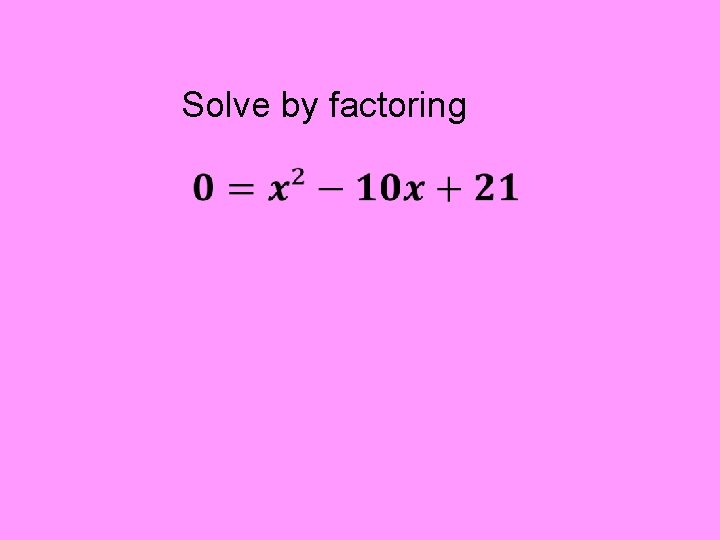Solve by factoring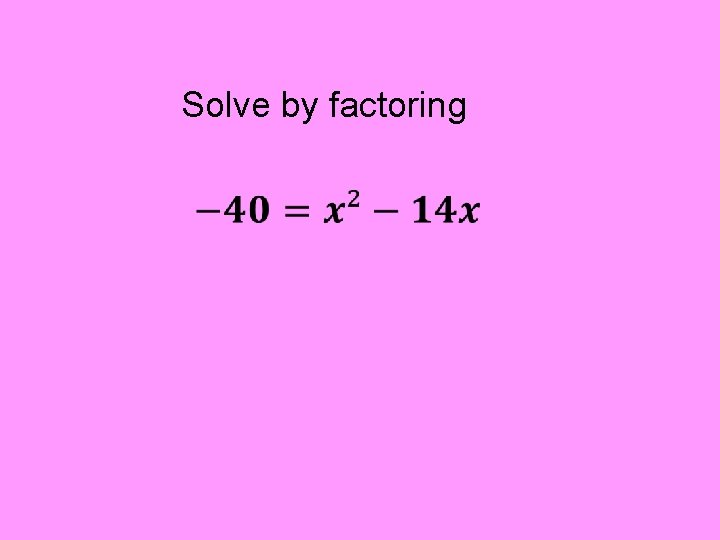Solve by factoring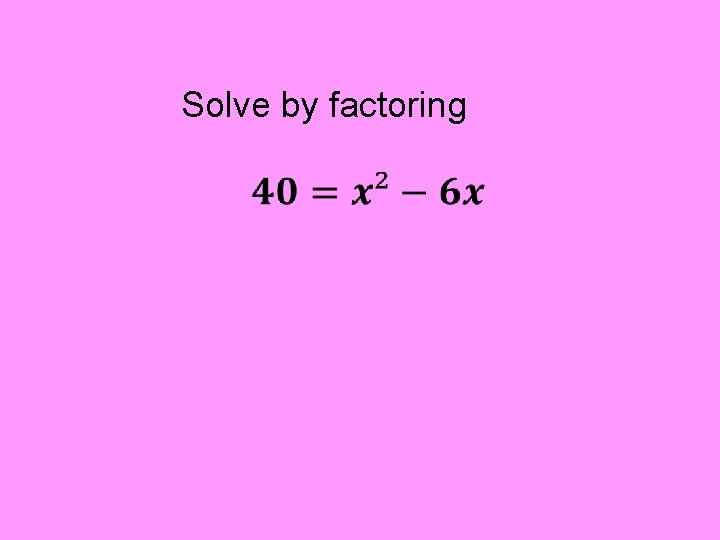Solve by factoring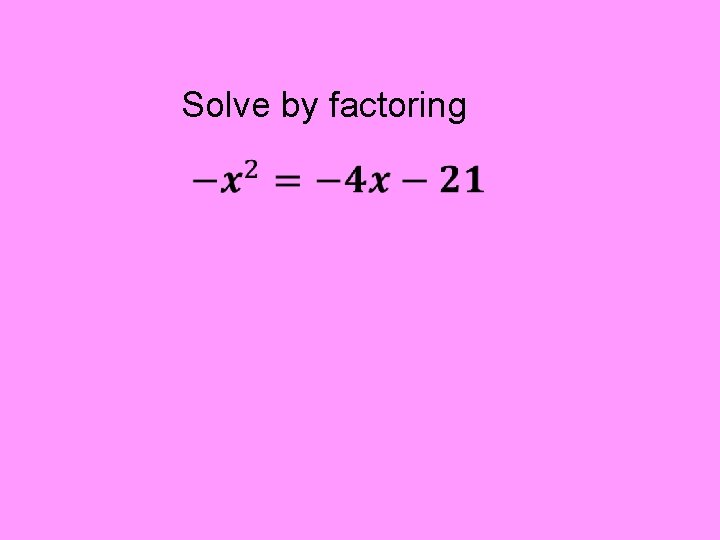Solve by factoring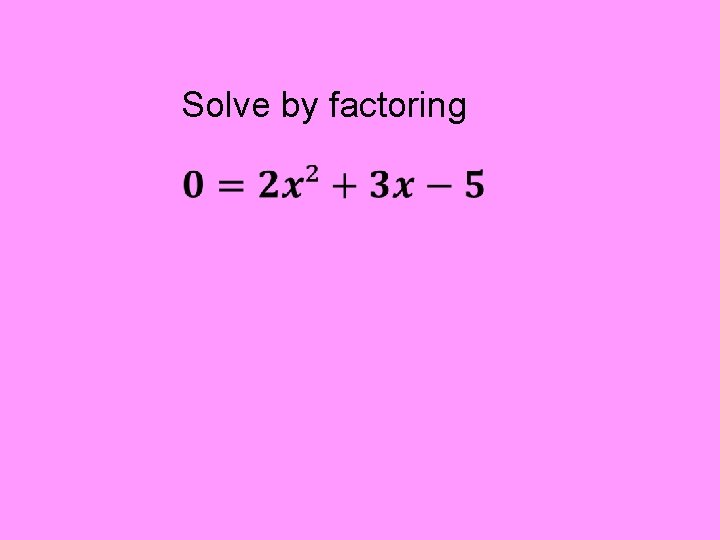Solve by factoringSolve by factoringSolve by factoring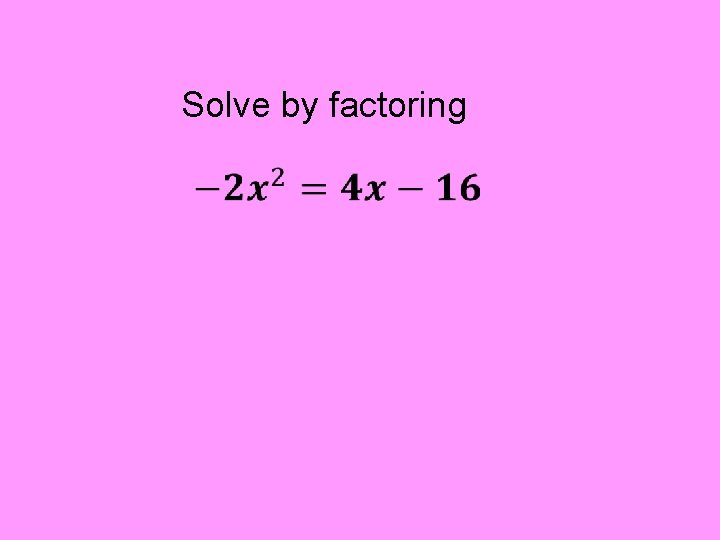Solve by factoring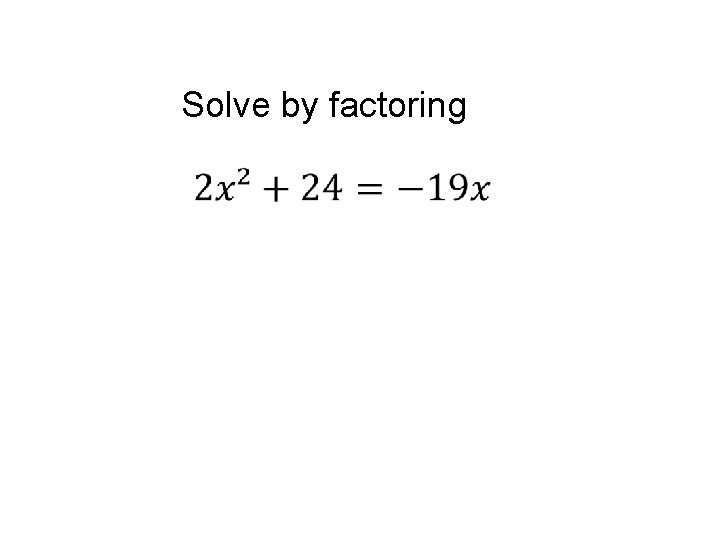Solve by factoringSolve by factoring.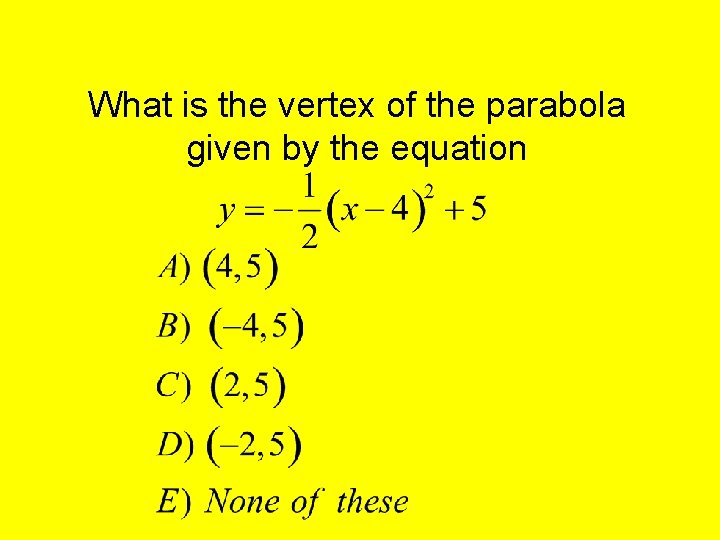What is the vertex of the parabola given by the equationWhich of the following statements is false about the graph of A) The parabola opens downward. B) The parabola is steeper than y = x 2. C) The line of symmetry is x = 4. D) The y-intercept is -3. E) None of these is false.Which of the following statements is false about the graph of A) B) C) D) E) The parabola opens downward. The line of symmetry is x = -1. The parabola is steeper than y = x 2. The y-intercept is -3. None of these is false.Which of the following is true about the graph of I. The graph contains the point (-6, -5). II. The slope of the graph is 2/3. III. The y-intercept of the graph is -1. A) I only B) II only C) III only D) I and II E) I and III F) II and III G) I, II, and III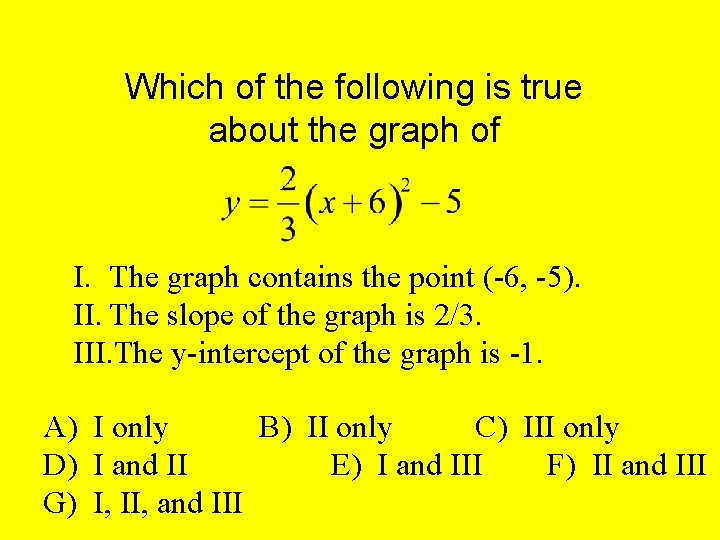Which of the following is true about the graph of I. The graph contains the point (-6, -5). II. The slope of the graph is 2/3. III. The y-intercept of the graph is -1. A) I only B) II only C) III only D) I and II E) I and III F) II and III G) I, II, and III